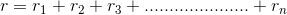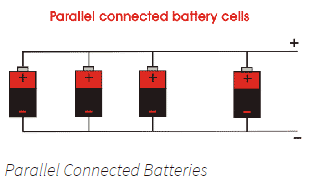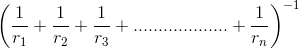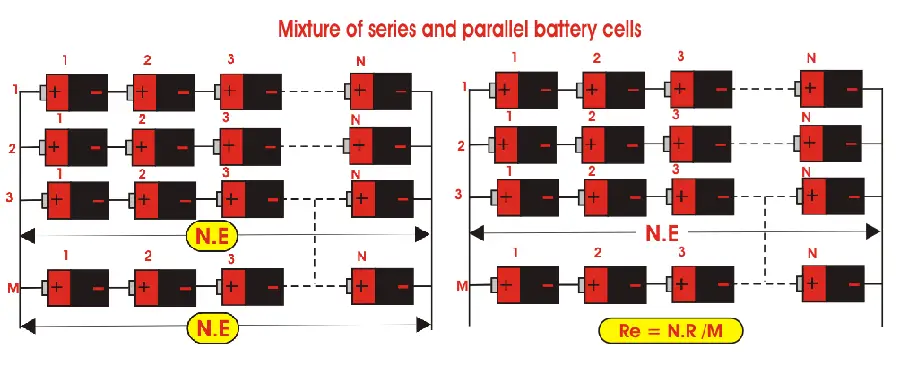# Batteries in Series and Batteries in Parallel

## Battery Cells

Battery is an electrical element where electrical potential is produced due to chemical reaction. Every electrochemical reaction has its limit of producing electric potential difference between two electrodes.
Battery cells are those where these electro-chemical reactions take place to produce the limited electric potential difference. For achieving desired electric potential difference across the battery terminals multiple numbers of cells are to be connected in series. Hence it can be concluded like that, a battery is a combination of several cells where a cell is a unit of a battery. For example, Nickel-cadmium battery cells normally develop about 1.2 V per cell while lead acid battery develop about 2 V per cell. So a 12 volt battery will have total 6 number of cells connected in series.

## EMF of Battery

If anyone just measures the electric potential difference between two terminals of a battery when load is not connected with the battery, he or she will get the voltage developed in the battery when there is no current flowing through it. This voltage is generally referred as electromotive force or emf of battery. It is also referred as no-load voltage of battery.

## Terminal Voltage of Battery

Terminal voltage of battery is the potential difference across its terminals when the current is being drawn from it. Actually when load is connected with the battery, there will be load current flowing through it. As a battery is an electrical equipment, it must have some electrical resistance inside it. Because of this internal resistance of battery , there will be some voltage drops across it. So, if any one measures the terminal voltage of the load i.e. terminal voltage of battery when load is connected, he or she will get the voltage which is less than emf of the battery by internal voltage drop of the battery.

If E is the emf or no-load voltage of the battery and V is the terminal voltage of load voltage of the battery, then E – V = internal voltage drop of the battery.
As per Ohm’s law, this internal voltage drop is nothing but the product of electrical resistance offered by the battery and the current flows through it.

## Internal Resistance of Battery

The entire resistance encountered by a current as if it flows through a battery from the negative terminal to the positive terminal is known as internal resistance of battery.

## Series Parallel Batteries

Battery cells can be connected in series, in parallel and as well as a mixture of both the series and parallel.

### Series Batteries

When in a battery, positive terminal of one cell is connected with the negative terminal of succeeding cell, then the cells are said to be series connected or simply series battery. Here, overall emf of the battery is algebraic sum of all individual cells connected in series. But overall discharged current of the battery does not exceed the discharged current of individual cells.If E is the overall emf of the battery combined by n number cells and E1, E2, E3, …………… En are the emfs of individual cells.Similarly, if r1, r2, r3, …………… rn are the internal resistances of individual cells, then the internal resistance of the battery will be equal to the sum of the internal resistance of the individual cells i.e.### Parallel Batteries

When positive terminals of all cells are connected together and similarly negative terminals of these cells are connected together in a battery, then the cells are said to be connected in parallel. These combinations are also referred as parallel batteries. If emf of each cell is identical, then the emf of the battery combined by n numbers of cells connected in parallel, is equal to the emf of each cell. The resultant internal resistance of the combination is,The current delivered by the battery is sum of currents delivered by individual cells.

### Mixed Grouping of Batteries or Series Parallel Batteries

As we said earlier, the cells in a battery can also be connected in mixture of both series and parallel. These combinations are some time referred as series parallel battery . A load can require both voltage and current more than that of an individual battery cell. For achieving the required load voltage, the desired numbers of battery cells can be combined in series and for achieving the required load current, desired numbers of these series combinations are connected in parallel. Let m, numbers of series, each containing n numbers of identical cells, are connected in parallel.Again assume emf of each cell is E and internal resistance of each cell is r. As n numbers of cells are connected in each series, the emf of each series as well as the battery will be nE. The equivalent resistance of the series is nr. As, m number of series connected in parallel equivalent internal resistance of that series and parallel battery is nr/m.

### Video Presentation about Series Parallel Batteries

Want To Learn Faster? 🎓
Get electrical articles delivered to your inbox every week.
No credit card required—it’s 100% free.